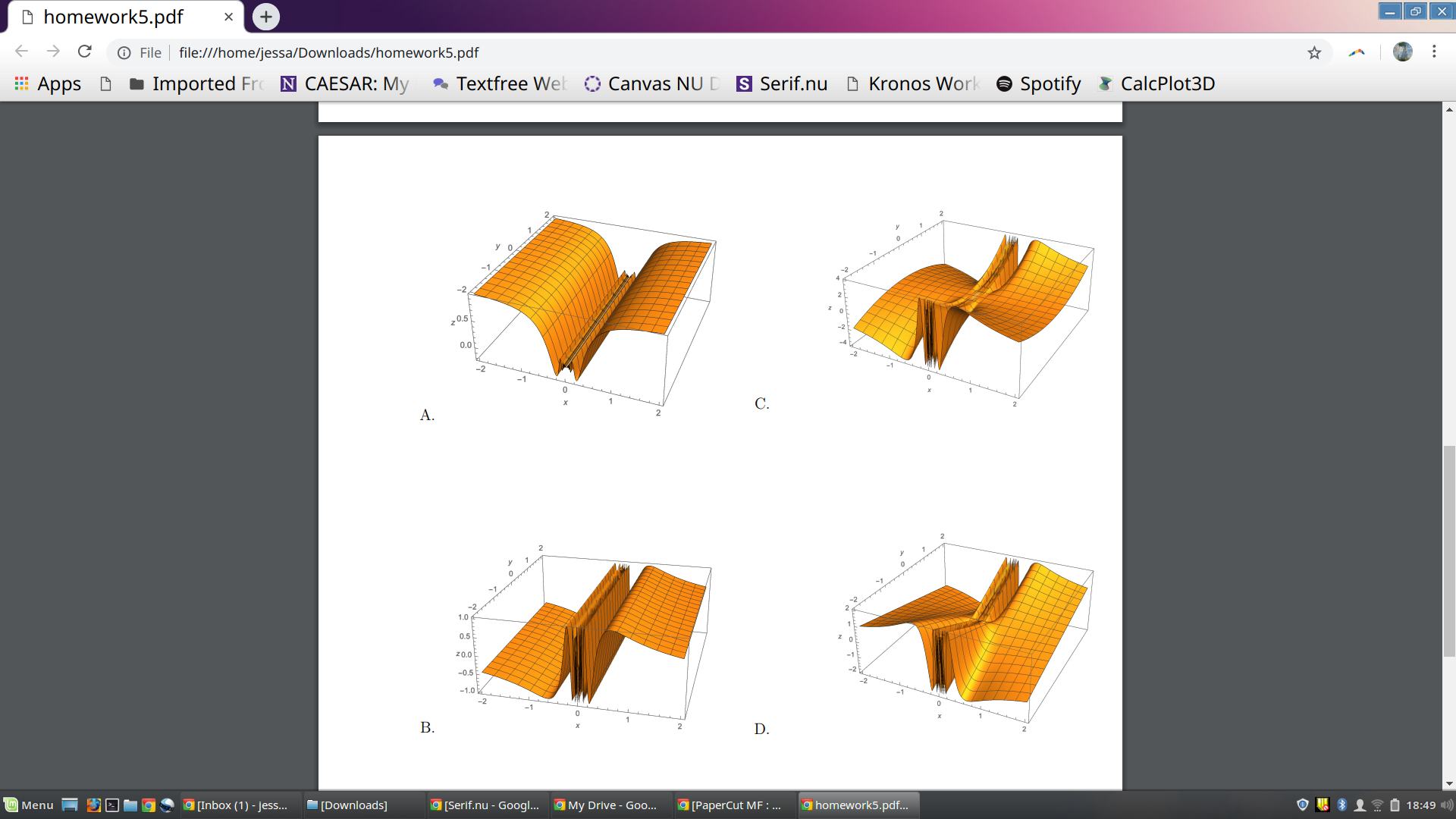D homework5.pdf x+← → ⓘ File file:///home/jessa/Downloads/homework5.pdfApps Imported Fre N CAESAR: My Textfree We ) Canvas NU S Serif.nu D Kronos WorkSpotifyCalcPlot3D0.50.01.00.5z0.0-0.5OMenu-圉囚一回包间[Inbox (1)-Jess...-[Downloads]serif.nu-GooglİMy Drive-Goo..PaperCut MF :g homework5.pdf

Question

Let f(x, y) = y sin(1/x).

(a) What is the domain of f?

I think it's all values of (x,y) where x is not 0

(b) What is the range of f; that is, which values does f(x, y) take for (x, y) in its domain?

I think it's all real numbers

(c) Determine the limit lim (x,y)→(0,0) y sin(1/x) and explain your answer.

(d) For c = 0 and c = 1, write an equation for the level curve f(x, y) = c, and draw them in the region where −2 ≤ x ≤ 2 and −2 ≤ y ≤ 2. Please use two different colors to indicate the two level curves, and feel free to use a graphing calculator to help you!

(e) Explain why lim (x,y)→(0,1) y sin(1/x) does not exist.

(f) From the surfaces drawn on the back of this page, determine the graph of f. (I attached as an image)help_outlineImage TranscriptioncloseD homework5.pdf x+ ← → ⓘ File file:///home/jessa/Downloads/homework5.pdf Apps Imported Fre N CAESAR: My Textfree We ) Canvas NU S Serif.nu D Kronos Work Spotify CalcPlot3D 0.5 0.0 1.0 0.5 z0.0 -0.5 OMenu- 圉囚一回包 间[Inbox (1)-Jess...-[Downloads] serif.nu-Googl İ My Drive-Goo.. PaperCut MF : g homework5.pdf fullscreen
Step 1

To analyze the given function of two variables.

Step 2

(a) Domain (f)]{(x,y): x not equal to 0}

Step 3

(b) Range of f= the set of al...

Want to see the full answer?

See Solution

Want to see this answer and more?

Our solutions are written by experts, many with advanced degrees, and available 24/7

See Solution
Tagged in

Calculus